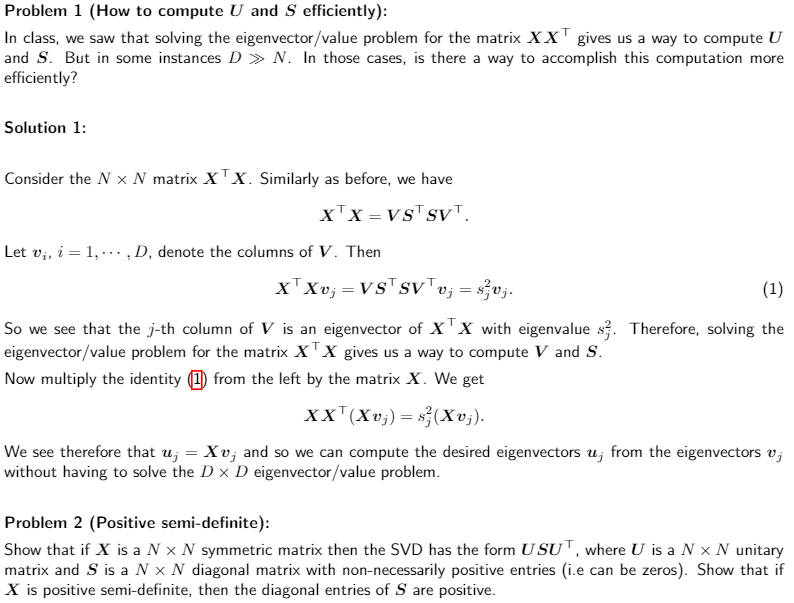### Lab 12 Problem 1 Compute U, S efficiently

1) possible typo In the solution
"Let $$v_i$$, i = 1, ..., N, denote the columns of V" instead of "i = 1, ..., D" since V is NxN matrix

2) I don't get how identity (1) is established, in particular why $$VS^TSV^Tv_j = s_j^2v_j$$Thank you!

Top comment

1) yes, I agree it is a typo in this case it should be N instead of D. In general it is the smallest of the two.

2) It is a direct implication of the identity $$X^\top X = V S^\top S V^\top$$ and the fact the columns of $$V$$ are orthonormal.

To be more precise $$X^\top X = V S^\top S V^\top$$ means $$X^\top X V = V S^\top S$$ which translates to $$X^\top X (v_1,\ldots,v_N) = V S^\top S(v_1,\ldots,v_N) = V (s_1^2 e_1,\ldots,s_N^2e_N)=(s_1^2 v_1,\ldots,s_N^2 v_N)$$
Where $$(e_i)_{i=1}^N$$ is the canonical basis of $$\mathcal{R}^N$$.

Page 1 of 1